# 人工智能导论 模糊逻辑

## Fuzzy Logic

Posted by R1NG on December 28, 2021 Viewed Times

# 模糊逻辑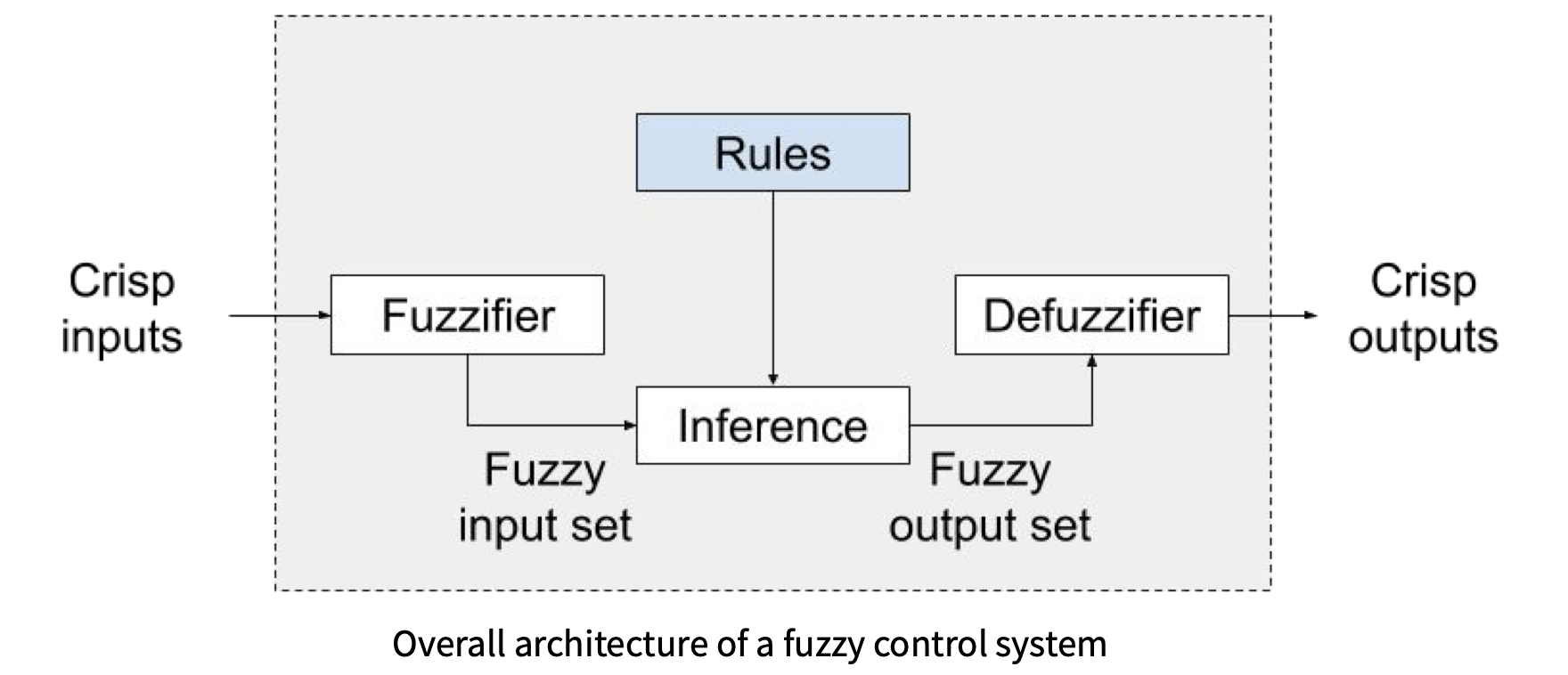## 2. 模糊化 (Fuzzification)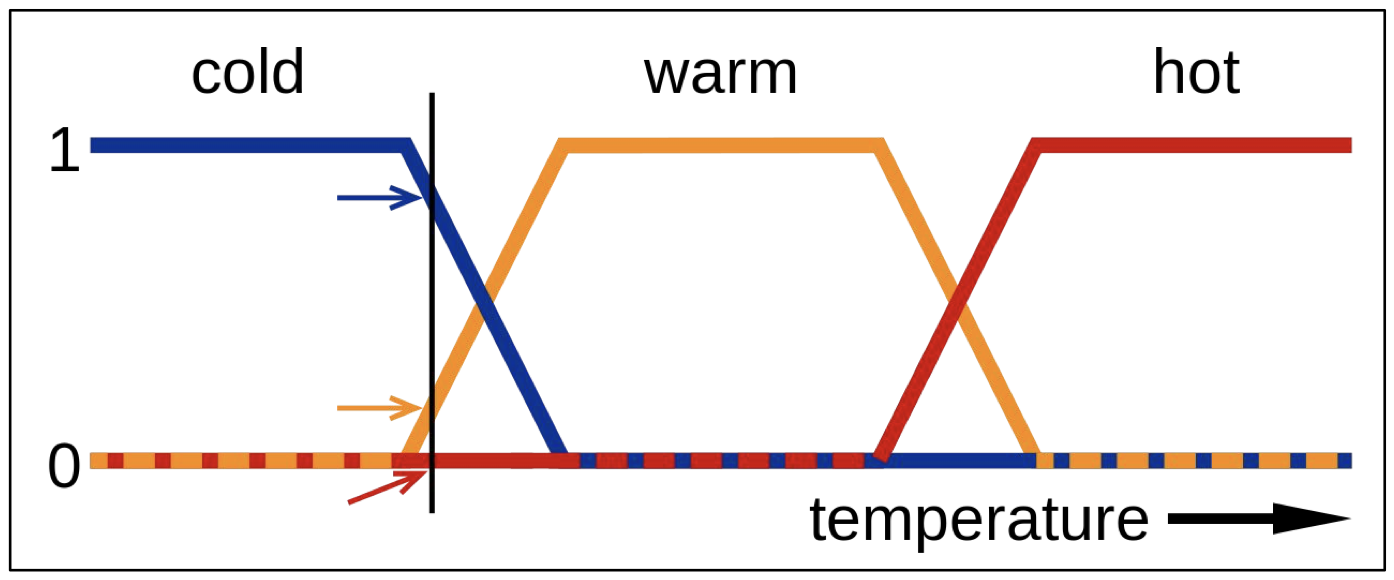## 3. 逻辑运算

1. 最小隶属法 (Conjunction):

$T(A \wedge B) = \text{min}(T(A), T(B))$
2. 最大隶属法 (Disjunction):

$T(A \vee B) = \text{max}(T(A), T(B))$
3. 取反 (Negation):

$T(\neg A) = 1-T(A)$

## 4. 决策规则

$\text{IF} ~<\text{antecedents}>~ \text{THEN} ~<\text{consequent}>~$

1. 给定前件规则 服务水平低或食材质量差, 限定结果 只给很少的小费;
2. 给定前件规则 服务水平比较好, 限定结果 只给一般水平的小费;
3. 给定前件规则 服务水平很高或食材质量很好, 限定结果 慷慨解囊.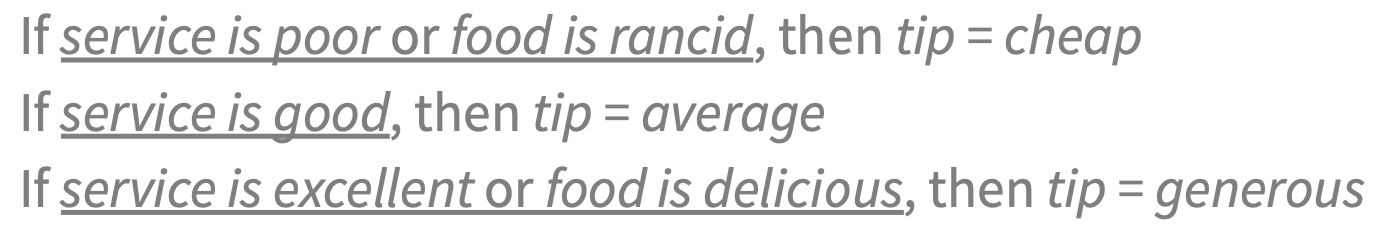1. 决策规则中的前件条件可以是某个由多个子条件组成的复合谓词公式, 如第一条规则中的前件条件实际上由两个子条件: 服务水低食材质量差 组成.
2. 决策规则中的前件条件可以是恰好由一个条件组成的复合谓词公式, 如第二条规则.
3. 决策规则中前件条件的形式可以是某个谓词公式的取反, 如第三条规则.

## 5. 推断和去模糊化

### Mamdami 模糊逻辑推理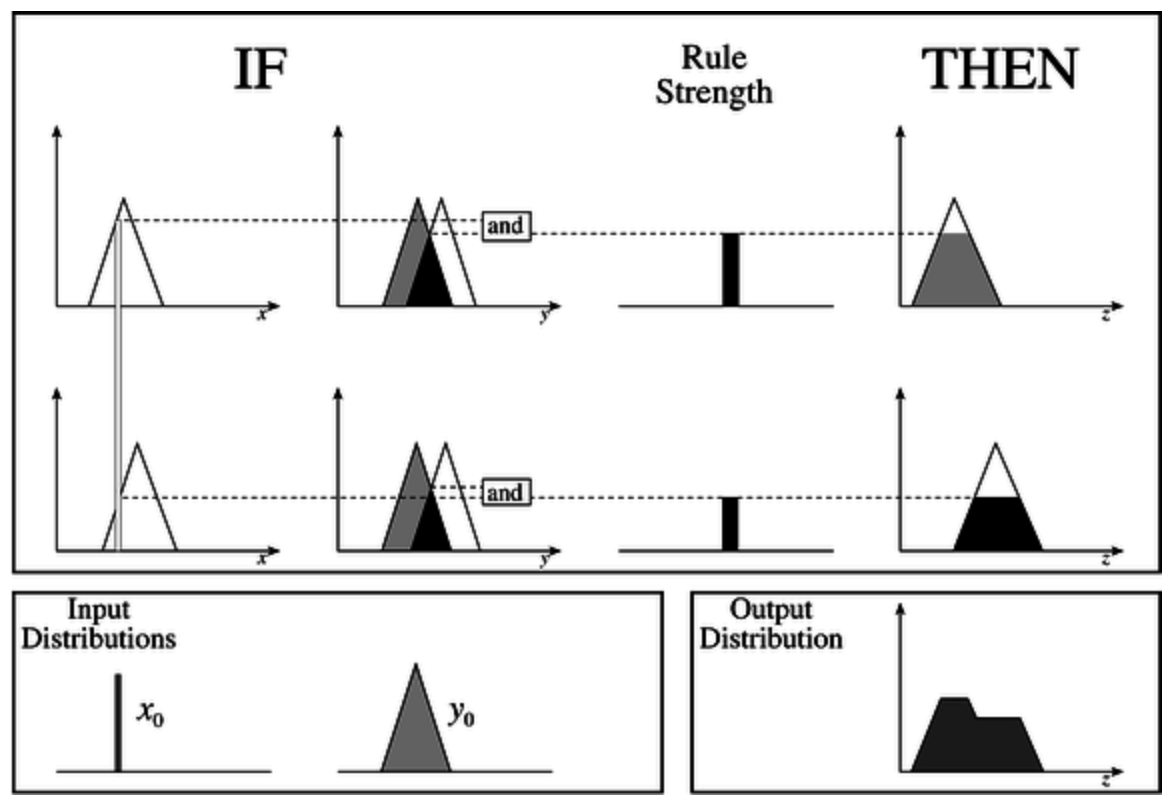1. 确定给定问题的所有模糊规则 (Fuzzy Rules)
2. 使用隶属函数将输入值模糊化.
3. 基于模糊规则将 已经被模糊化的输入 结合, 进而计算出该规则在给定模糊输入下对应的 规则强度 (Rule Strength).
4. 结合上一步中计算出的 规则强度给定规则的输出隶属函数, 得出基于当前的模糊输入下该规则的 结果 (Consequence).
5. 将来自所有规则的结果相结合, 从而得出输出分布 (Output Distribution).

1. 在第四步中, 结果 是通过将 给定规则的输出隶属函数规则强度 的高度上 推平 得到的: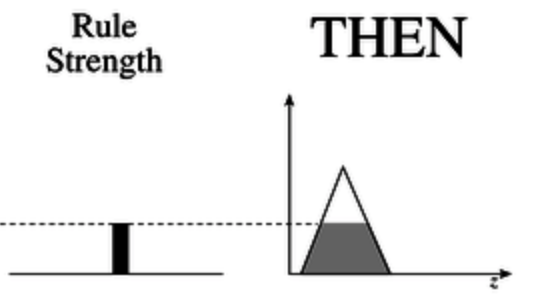1. 在第五步中, 绝大多数情况下 输出分布在定义域上任何点的值 都是 全体规则的输出隶属函数的析取 (也就是取并):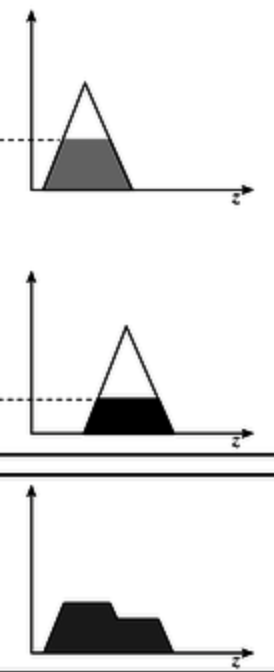$z = \frac{\sum_{i=1}^{q} Z_i \cdot u_c(Z_i)}{\sum_{i=1}^{q} u_c(Z_i)}$

### Sugeno 模糊逻辑推理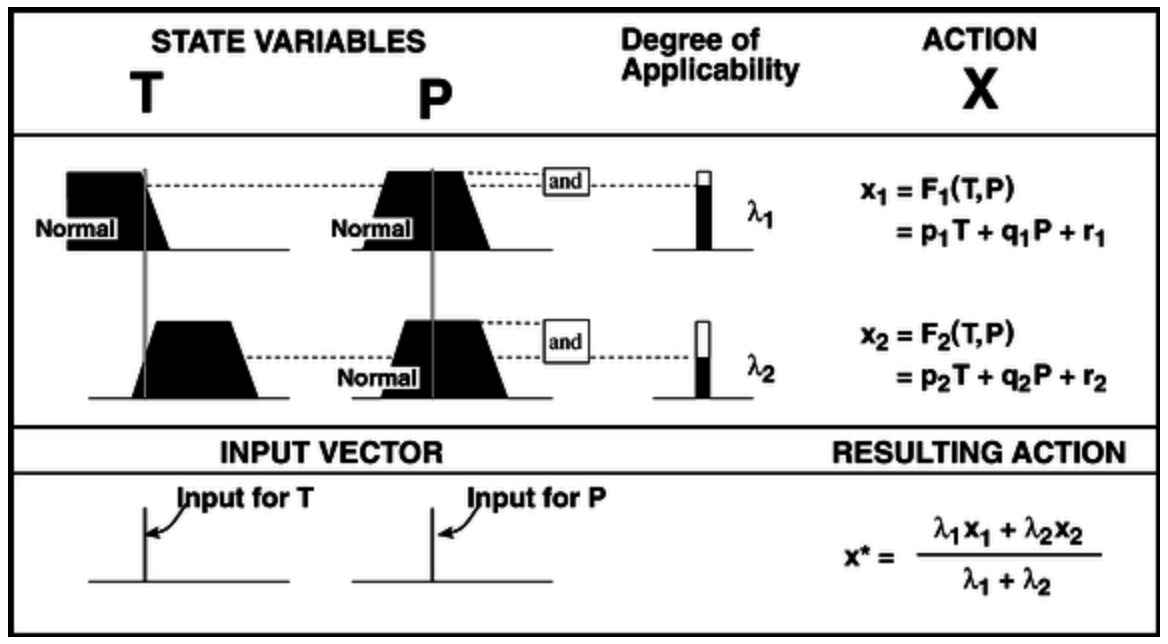Sugeno 模糊逻辑推理Mamdami 模糊逻辑推理 的流程基本类似, 主要区别在前者 不以隶属函数作为输出, 其输出是通过以 每条规则的输出隶属函数取极值时对应的 $x$ 坐标 为系数, 和 每条规则在给定的模糊输入下算出的, 对应的规则强度加权平均 得到的.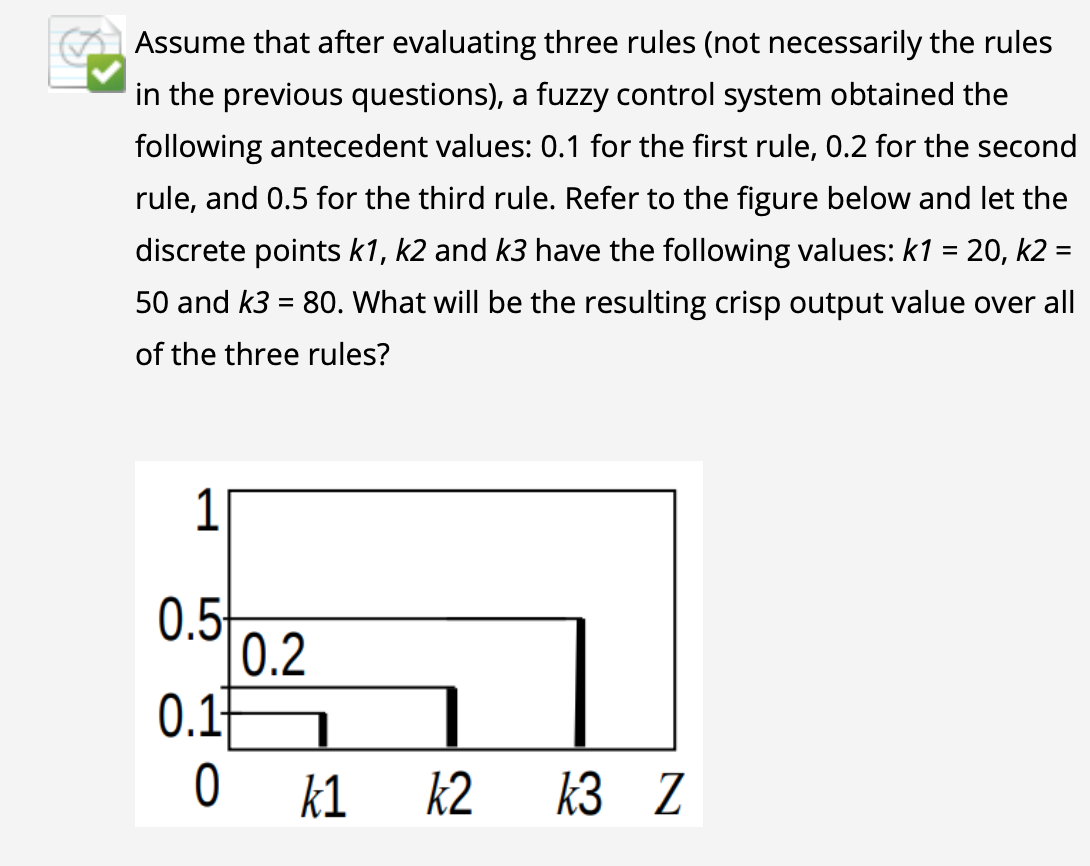$\text{ans} = \frac{k_1 \cdot \lambda_1 + k_2 \cdot \lambda_2 + k_3 \cdot \lambda_3}{\lambda_1 + \lambda_2 + \lambda_3} = \frac{0.1 \cdot 20 + 0.2 \cdot 50 + 0.5 \cdot 80}{0.1 + 0.2 + 0.5} = 65.$

Reference: